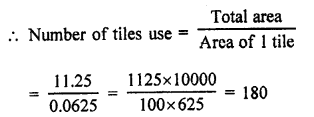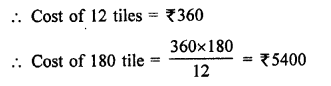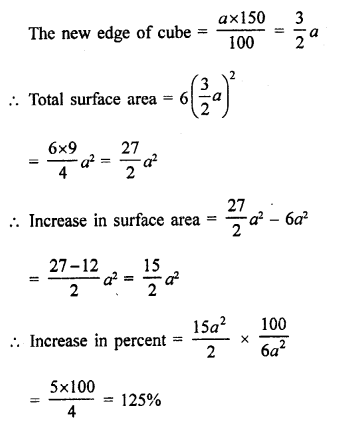• +91 9971497814
• info@interviewmaterial.com

# RD Chapter 18- Surface Areas and Volume of a Cuboid and Cube Ex-18.1 Interview Questions Answers

### Related Subjects

Question 1 : Find the lateral surface area and total surface area of a cuboid of length 80 cm, breadth 40 cm and height 20 cm.

Length of cuboid (l) = 80 cm
Height (h) = 20 cm
(i) ∴ Lateral surface area = 2h(l + b)
= 2 x 20(80 + 40) cm²
= 40 x 120 = 4800 cm²
(ii) Total surface area = 2(lb + bh + hl)
= 2(80 x 40 + 40 x 20 + 20 x 80) cm²
= 2(3200 + 800 + 1600) cm²
= 5600 x 2 = 11200 cm²

Question 2 : Find the lateral surface area and total surface area of a cube of edge 10 cm.

Edge of cube (a) = 10 cm
(i) ∴ Lateral surface area = 4a²
= 4 x (10)² = 4 x 100 cm²= 400 cm²
(ii) Total surface area = 6a² = 6 x(10)² cm²
= 6 x 100 = 600 cm²

Question 3 : Find the ratio of the total surface area and lateral surface area of a cube.

Let a be the edge of the cube, then Total surface area = 6a2²
and lateral surface area = 4a²
Now ratio between total surface area and lateral surface area = 6a² : 4a² = 3 : 2

Question 4 : Mary wants to decorate her Christmas tree. She wants to place the tree on a wooden block covered with coloured paper with picture of Santa Claus on it. She must know the exact quantity of paper to buy for this purpose. If the box has length, breadth and height as 80 cm, 40 cm and 20 cm respectively. How many square sheets of paper of side 40 cm would she require?   [NCERT]

Length of box (l) = 80 cm
and height (h) = 20 cm
∴ Total surface area = 2(lb + bh + hl)
= 2[80 x 40 + 40 x 20 + 20 x 80] cm²
= 2[3200 + 800 + 1600] cm² = 2 x 5600 = 11200 cm²
Size of paper sheet = 40 cm
∴ Area of one sheet = (40 cm)² = 1600 cm²
∴ No. of sheets required for the box = 11200 = 1600 = 7 sheets

Question 5 : The length, breadth and height of a room are 5 m, 4 m and 3 m respectively. Find the cost of white washing the walls of the room and the ceiling at the rate of ₹7.50 m².

Length of a room (l) = 5m
and height (h) = 3 m
∴ Area of 4 walls = 2(l + b) x h
= 2(5 + 4) x 3 = 6 x 9 = 54 m²
and area of ceiling = l x b = 5 x 4 = 20 m²
∴ Total area = 54 + 20 = 74 m2
Rate of white washing = 7.50 per m²
∴ Total cost = ₹74 x 7.50 = ₹555

Question 6 : Three equal cubes are placed adjacently in a row. Find the ratio of total surface area of the new cuboid to that of the sum of the surface areas of the three cubes.

Let each side of a cube = a cm
Then surface area = 6a² cm²
and surface area of 3 such cubes = 3 x 6a² = 18a² cm²
By placing three cubes side by side we get a cuboid whose,
Length (l) = a x 3 = 3a
Height (h) = a
∴ Total surface area = 2(lb + bh + hf)
= 2[3a x a+a x a+a x 3a] cm²
= 2[3a² + a² + 3a²] = 14 a²
∴ Ratio between their surface areas = 14a² : 18a² = 7 : 9

Question 7 : A 4 cm cube is cut into 1 cm cubes. Calculate the total surface area of all the small cubes.

Side of cube = 4 cm
But cutting into 1 cm cubes, we get = 4 x 4 x 4 = 64
Now surface area of one cube = 6 x (1)²
= 6 x 1=6 cm²
and surface area of 64 cubes = 6 x 64 cm² = 384 cm²

Question 8 : The length of a hall is 18 m and the width 12 m. The sum of the areas of the floor and the flat roof is equal to the sum of the areas of the four walls. Find the height of the hall.

Let h be the height of the room
Length (l) = 18 m
and width (b) = 12 m
Now surface area of floor and roof = 2 x lb = 2 x 18 x 12 m²
= 432 m²
and surface area of 4-walls = 2h (l + b)
= 2h(18 + 12) = 2 x 30h m² = 60h m²
∵ The surface are of 4-walls and area of floor and roof are equal
∴ 60h = 432
⇒ h = 432/60 = 72/10 m
∴ Height = 7.2m

Question 9 : Hameed has built a cubical water tank with lid for his house, with each other edge 1.5m long. He gets the outer surface of the tank excluding the base, covered with square tiles of side 25 cm. Find how much he would spend for the tiles, if the cost of tiles is ₹360 per dozen. [NCERT]

Edge of cubical tank = 1.5 m
∴ Area of 4 walls = 4 (side)² = 4(1.5)² m² = 4 x 225 = 9 m²
Area of floor = (1.5)² = 2.25 m²
∴ Total surface area = 9 + 2.25 = 11.25 m²
Edge of square tile = 25 m = 0.25 m²
∴ Area of 1 tile = (0.25)2 = .0625 m²Question 10 : Each edge of a cube is increased by 50%. Find the percentage increase in the surface area of the cube.

Let edge of a cube = a
Total surface area = 6a2
By increasing edge at 50%,Todays Deals### RD Chapter 18- Surface Areas and Volume of a Cuboid and Cube Ex-18.1 Contributorskrishan

Name:
Email:

# Latest News# 9000 interview questions in different categories